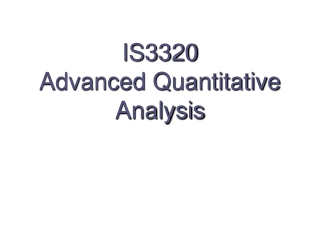DownloadDownload PresentationTélécharger la présentation- - - - - - - - - - - - - - - - - - - - - - - - - - - E N D - - - - - - - - - - - - - - - - - - - - - - - - - - -
##### Presentation Transcript

2. Course Objectives This course aims to: • Describe the quantitative analysis approach and it is applications in a real situation. • illustrate the use of modeling in quantitative analysis. • Use the simplex method to solve linear programming problems.

3. Course Objectives Tackle a wide variety of problems by simulation. Structure special LP problems using the transportation and assignment models Understand the difference between LP and integer programming Understand and solve the three types of integer programming problems

4. Course Syllabus 1) Simplex method: • Introduction • How to Set Up the Initial Simplex Solution • Simplex Solution Procedures • The Second Simplex Tableau • Developing the Third Tableau • Review of Procedures for Solving LP Maximization Problems • Surplus and Artificial Variables

5. Course Syllabus 2) Advanced decision analysis: • Develop accurate and useful decision tree. • Understand the importance and use of utility theory in decision making.

6. Course Syllabus 3) Markov analysis: • introduction. • Regular markov chain. • Absorbing markov chain. • Steady state of markov chain.

7. Course Syllabus 4) Integer programming: • Difference between linear programming and integer programming. • Solve integer programming problems.

8. Course Syllabus 5) Transportation problem: • Introduction • Setting Up a Transportation Problem • Developing an Initial Solution, northwest Corner Rule • Stepping-Stone Method: Finding a Least-Cost Solution

9. Course Syllabus 6) Goal programming: • Introduction. • Solve goal programming problems.

10. Course Syllabus 7) Simulation modelling: • Introduction • Advantages and Disadvantages of Simulation • Monte Carlo Simulation • Simulation of a Queuing Problem • Two Other Types of Simulation • Verification and Validation

11. Course Syllabus 8) Dynamic programming: • How to solve shortest path by dynamic programming

12. Text Book • Render, Stair, and Hanna (2009). Quantitative Analysis for Management, Tenth Edition, Prentice-Hall, Inc.

13. Assessment Tasks for Students During the Semester • 3 Hours final exam 50 Marks • 1 Hour first test 15 Marks • 1 Hour Second Test 15 Marks • 2 Quizzes 10 Marks • 2 Assignments 10 Marks • Exam1 16/6/1438 15/3/2017 • Exam2 29/7/1438 26/4/2017